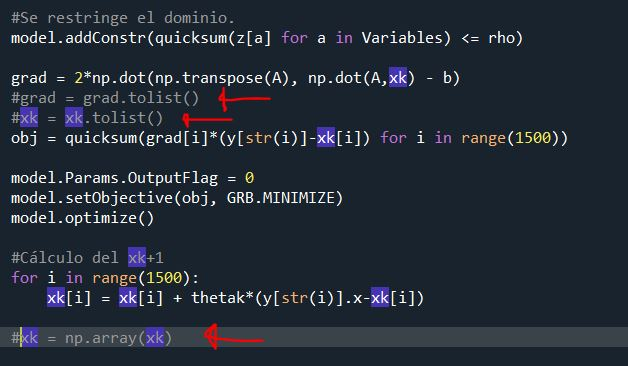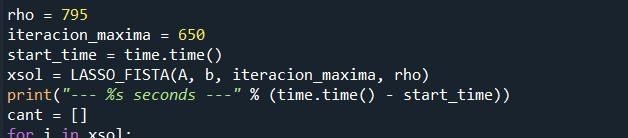•Gurobi Staff

Hi Cris,

Could you post the code snippet, in best case a self contained example, of how you perform the multiplication of the array/list and your variables?

Best regards,
Jaromił

•Sure. I used the Frank-Wolfe algorithm. This is my code.

from parámetros import *from gurobipy import Model, GRB, quicksumimport timeimport matplotlibimport matplotlib.pyplot as pltdef LASSO_FISTA(A, b, iteracion_maxima, rho):    m, n = A.shape    xk = np.zeros(n,)  #Vector x_k inicial    thetak = 1    norma_b = np.linalg.norm(b)    Variables = []    for i in range(1500):        Variables.append(str(i))    print("\n\n**********    METODO FISTA    *********\n")    print("ITERACION     VALOR OBJ      ERROR AJUSTE  ||x||_1")    for iteracion in range(iteracion_maxima):         model = Model("Modelo")        y = model.addVars(Variables,vtype=GRB.CONTINUOUS,lb= -GRB.INFINITY, ub= GRB.INFINITY, name="y")        z = model.addVars(Variables, lb = 0, vtype=GRB.CONTINUOUS, name="z") #Variable auxiliar        model.update()        model.addConstrs((y[a] <= z[a] for a in Variables),name = "abs")        model.addConstrs((-z[a] <= y[a] for a in Variables), name="abs")        #Se restringe el dominio.         model.addConstr(quicksum(z[a] for a in Variables) <= rho)        grad = 2*np.dot(np.transpose(A), np.dot(A,xk) - b)        obj = quicksum(grad[i]*(y[str(i)]-xk[i]) for i in range(1500))                       model.Params.OutputFlag = 0        model.setObjective(obj, GRB.MINIMIZE)        model.optimize()        #Cálculo del xk+1        for i in range(1500):            xk[i] = xk[i] + thetak*(y[str(i)].x-xk[i])                #Actualización valores con el nuevo valor de xk        thetak = 2/(2+iteracion)        valor_optimo = np.linalg.norm(np.dot(A, xk) - b)**2        error = np.linalg.norm(np.dot(A, xk) - b)/norma_b        n1 = np.linalg.norm(xk, 1)        retorno_en_pantalla = [iteracion, valor_optimo, error, n1]        sucesion_error_.append(error)        sucesion_norma_1.append(n1)        iteraciones_.append(iteracion)        print("%12.6f %12.6f %12.6f %12.6f" % (retorno_en_pantalla,retorno_en_pantalla,retorno_en_pantalla,retorno_en_pantalla))    return xk
•The list option was. I convert the gradient and xk vector to a list, multiply and after that transform the xk to an array vector to repeat the process

        grad = 2*np.dot(np.transpose(A), np.dot(A,xk) - b)        grad = grad.tolist()        xk = xk.tolist()        obj = quicksum(grad[i]*(y[str(i)]-xk[i]) for i in range(1500))        model.Params.OutputFlag = 0        model.setObjective(obj, GRB.MINIMIZE)        model.optimize()        for i in range(1500):            xk[i] = xk[i] + thetak*(y[str(i)].x-xk[i])                 xk = np.array(xk)
•Gurobi Staff

Hi Cris,

Which functions/loops did you time in your first message? Was it only the $$\texttt{obj}$$ computation

obj = quicksum(grad[i]*(y[str(i)]-xk[i]) for i in range(1500))

Best regards,
Jaromił

•I timed the execution of the entire LASSO_FISTA(A, b, iteracion-maxima, rho)  function. But, I think that it is equivalent to time just

obj = quicksum(grad[i]*(y[str(i)]-xk[i]) for i in range(1500))

because It is the only change in the code.

The red lines comented are the only difference between both codes.Timer•Gurobi Staff

Hi Cris,

In the following I dropped $$\texttt{xk}$$ as all elements are zero and it makes the answer a bit easier.

The time consuming operation is not the $$\texttt{quicksum}$$ but rather the conversion of

grad[i]*w[i] for i in range(n)

to a list takes the most time. I tested it via

n = 15000grad = np.random.rand(n)w = m.addVars(n, vtype=GRB.CONTINUOUS,lb= -GRB.INFINITY, ub= GRB.INFINITY, name="w")start = time.time()l = list(grad[i]*w[i] for i in range(n))end = time.time()print("list computation took: "+str(end-start)+" seconds")

In general, we recommend to set the objective coefficients directly in the variable definition via

w = m.addVars(n, obj=grad ,vtype=GRB.CONTINUOUS,lb= -GRB.INFINITY, ub= GRB.INFINITY, name="w")

to avoid such bottlenecks.

Best regards,
Jaromił

•I think I understand. I am not sure but when you multiply a var by a "vector", in this case an array vector, Gurobi takes the result as a list, where each component of the result It is an element in the list, isn't it??

•Gurobi Staff

Hi Cris,

Yes, since Gurobi's quicksum takes a list as argument, Python first converts the

grad[i]*w[i] for i in range(n)

part into a list, where each $$\texttt{grad[i]}$$ is an array element and $$\texttt{w[i]}$$ is a tupledict element (as it was constructed via addVars). After the list is generated, then each component $$\texttt{grad[i]*w[i]}$$ is a list element.

Best regards,
Jaromił

•Perfect. I understand. Thanks for your help. I would like to know where I could find more information about details like quicksums use list as an argument and improve my codes??

Best Regards.

Cris

•Gurobi Staff

Hi Cris,

The best way would be to check the documentation of each such specific function and see what are the input arguments. For the Python API, you can expect that $$\texttt{list}$$ is most likely the input argument, since arrays require the $$\texttt{numpy}$$ module.

Best regards,
Jaromił